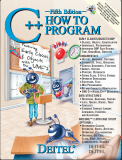Back to www.deitel.comISBN: 0-13-185757-6 © 2005 pages: 1500 Buy the Book!This tutorial introduces C++ exception handling. An exception is an indication of a problem that occurs during a program's execution. The name "exception" implies that the problem occurs infrequently—if the "rule" is that a statement normally executes correctly, then the "exception to the rule" is that a problem occurs. The techniques presented in this tutorial enable programmers to write robust, fault-tolerant programs that are able to deal with problems that may arise, and continue executing or terminate gracefully. We demonstrate exception-handling techniques with an example in which a function generates an exception when an attempt is made to divide by zero. The program catches this exception, issues an error message, then continues normal execution. This tutorial is intended for students and professionals who are already familiar with building C++ classes.

[Note: This tutorial is an excerpt (Section 16.3) of Chapter 16, Exception Handling, from our textbook C++ How to Program, 5/e. These tutorials may refer to other chapters or sections of the book that are not included here. Permission Information: Deitel, Harvey M. and Paul J., C++ HOW TO PROGRAM, ©2005, pp.812-818. Electronically reproduced by permission of Pearson Education, Inc., Upper Saddle River, New Jersey.]

Defining an Exception Class to Represent the Type of Problem That Might Occur

Figure 16.1 defines class `DivideByZeroException` as a derived class of Standard Library class `runtime_error` (defined in header file `<stdexcept>`). Class `runtime_error`—a derived class of Standard Library class `exception` (defined in header file <exception>)—is the C++ standard base class for representing runtime errors. Class `exception` is the standard C++ base class for all exceptions. (Section 16.13 discusses class `exception` and its derived classes in detail.) A typical exception class that derives from the `runtime_error` class defines only a constructor (e.g., lines 12–13) that passes an error-message string to the base-class `runtime_error` constructor. Every exception class that derives directly or indirectly from `exception` contains the `virtual` function `what`, which returns an exception object’s error message. Note that you are not required to derive a custom exception class, such as `DivideByZeroException`, from the standard exception classes provided by C++. However, doing so allows programmers to use the `virtual` function `what` to obtain an appropriate error message. We use an object of this `DivideByZeroException` class in Fig. 16.2 to indicate when an attempt is made to divide by zero.

 ``` 1 // Fig. 16.1: DivideByZeroException.h 2 // Class DivideByZeroException definition. 3 #include // stdexcept header file contains runtime_error 4 using std::runtime_error; // standard C++ library class runtime_error 5 6 // DivideByZeroException objects should be thrown by functions 7 // upon detecting division-by-zero exceptions 8 class DivideByZeroException : public runtime_error 9 { 10 public: 11 // constructor specifies default error message 12 DivideByZeroException::DivideByZeroException() 13 : runtime_error( "attempted to divide by zero" ) {} 14 }; // end class DivideByZeroException ```

Fig. 16.1 Class `DivideByZeroException` definition.

 ``` 1 // Fig. 16.2: Fig16_02.cpp 2 // A simple exception-handling example that checks for 3 // divide-by-zero exceptions. 4 #include 5 using std::cin; 6 using std::cout; 7 using std::endl; 8 9 #include "DivideByZeroException.h" // DivideByZeroException class 10 11 // perform division and throw DivideByZeroException object if 12 // divide-by-zero exception occurs 13 double quotient( int numerator, int denominator ) 14 { 15 // throw DivideByZeroException if trying to divide by zero 16 if ( denominator == 0 ) 17 throw DivideByZeroException(); // terminate function 18 19 // return division result 20 return static_cast< double >( numerator ) / denominator; 21 } // end function quotient 22 23 int main() 24 { 25 int number1; // user-specified numerator 26 int number2; // user-specified denominator 27 double result; // result of division 28 29 cout << "Enter two integers (end-of-file to end): "; 30 31 // enable user to enter two integers to divide 32 while ( cin >> number1 >> number2 ) 33 { 34 // try block contains code that might throw exception 35 // and code that should not execute if an exception occurs 36 try 37 { 38 result = quotient( number1, number2 ); 39 cout << "The quotient is: " << result << endl; 40 } // end try 41 42 // exception handler handles a divide-by-zero exception 43 catch ( DivideByZeroException ÷ByZeroException ) 44 { 45 cout << "Exception occurred: " 46 << divideByZeroException.what() << endl; 47 } // end catch 48 49 cout << "\nEnter two integers (end-of-file to end): "; 50 } // end while 51 52 cout << endl; 53 return 0; // terminate normally 54 } // end main ```
Fig. 16.2  Exception-handling example that throws exceptions on attempts to divide by zero.

Output

 ``` Enter two integers (end-of-file to end): 100 7 The quotient is: 14.2857 Enter two integers (end-of-file to end): 100 0 Exception occurred: attempted to divide by zero Enter two integers (end-of-file to end): ^Z ```

Fig. 16.2 Exception-handling example that throws exceptions on attempts to divide by zero.

Additional Pages in this Tutorial: Page 1 | 2 | 3 | 4
Tutorial Index